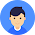# What is a union

## How to define a union?

We use the `union` keyword to define unions. Here's an example:
```
`union car`
`{`
`  char name;`
`  int price;`
`};`

```
The above code defines a derived type `union car`.

## Create union variables

When a union is defined, it creates a user-defined type. However, no memory is allocated. To allocate memory for a given union type and work with it, we need to create variables.
Here's how we create union variables.
```
`union car`
`{`
`  char name;`
`  int price;`
`};`

`int main()`
`{`
`  union car car1, car2, *car3;`
`  return 0;`
`}`

```
Another way of creating union variables is:
```
`union car`
`{`
`  char name;`
`  int price;`
`} car1, car2, *car3;`

```
In both cases, union variables car1car2, and a union pointer car3 of `union car` type are created.

### Access members of a union

We use the `.` operator to access members of a union. To access pointer variables, we use also use the `->` operator.
In the above example,
• To access price for `car1``car1.price` is used.
• To access price using `car3`, either `(*car3).price` or `car3->price` can be used.

## Difference between unions and structures

Let's take an example to demonstrate the difference between unions and structures:
```
`#include <stdio.h>`
`union unionJob`
`{`
`   //defining a union`
`   char name;`
`   float salary;`
`   int workerNo;`
`} uJob;`

`struct structJob`
`{`
`   char name;`
`   float salary;`
`   int workerNo;`
`} sJob;`

`int main()`
`{`
`   printf("size of union = %d bytes", sizeof(uJob));`
`   printf("\nsize of structure = %d bytes", sizeof(sJob));`
`   return 0;`
`}`

```
Output
```size of union = 32
size of structure = 40```
```
```

1.2.3.